# Quantum Mechanics Wavefunctions and Quantum Wells Quantum Mechanics

• Slides: 14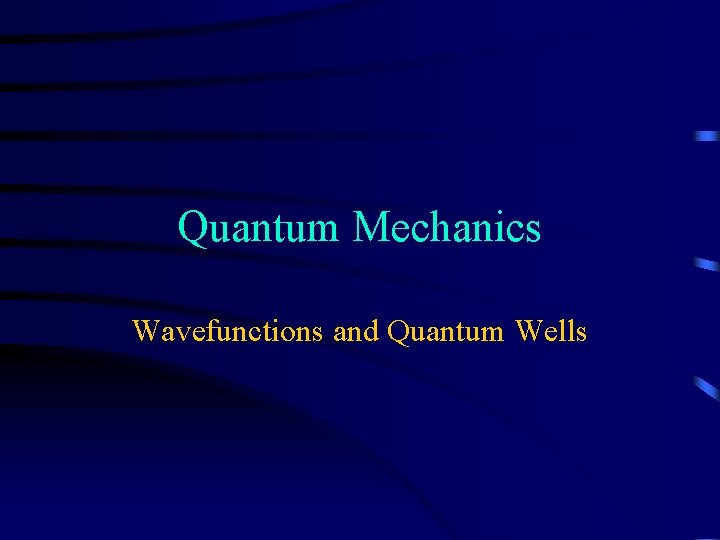Quantum Mechanics Wavefunctions and Quantum Wells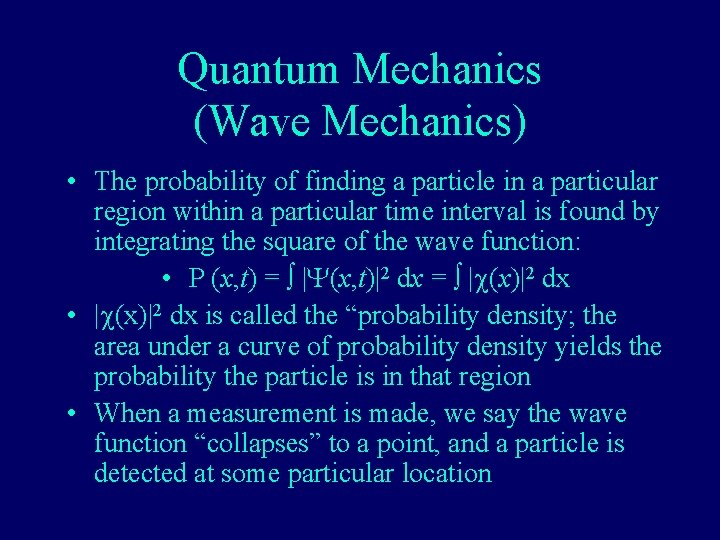Quantum Mechanics (Wave Mechanics) • The probability of finding a particle in a particular region within a particular time interval is found by integrating the square of the wave function: • P (x, t) = |Y(x, t)|2 dx = |c(x)|2 dx • |c(x)|2 dx is called the “probability density; the area under a curve of probability density yields the probability the particle is in that region • When a measurement is made, we say the wave function “collapses” to a point, and a particle is detected at some particular location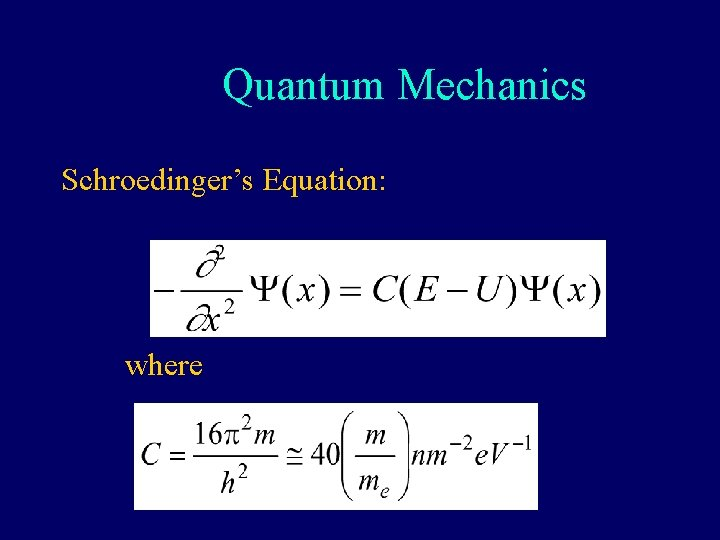Quantum Mechanics Schroedinger’s Equation: whereApplication: particle in a box • If a particle is confined to a region by infinitelyhigh walls, the probability of finding it outside that region is zero. • Since nature is generally continuous (no instantaneous changes), the probability of finding it at the edges of the region is zero. • The position-dependent solution to the Schrödinger equation for this case has the form of a sine function: c(x) = B sin (npx/a) (a=well width)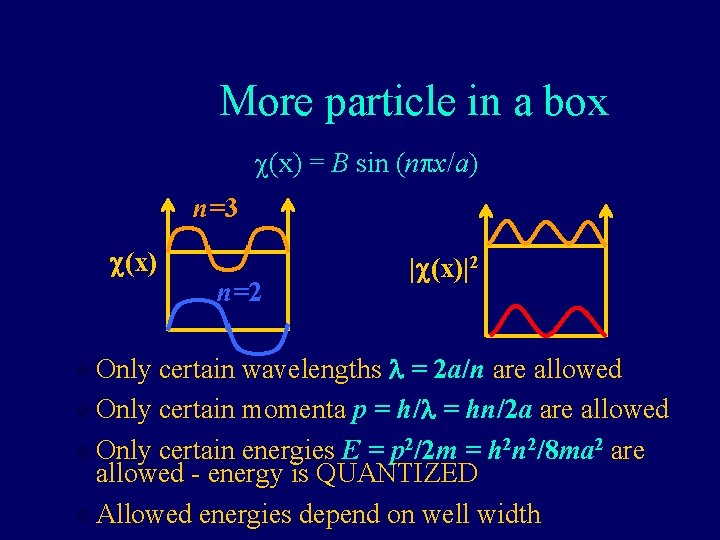More particle in a box c(x) = B sin (npx/a) n=3 c(x) n=2 |c(x)|2 certain wavelengths l = 2 a/n are allowed v Only certain momenta p = h/l = hn/2 a are allowed v Only certain energies E = p 2/2 m = h 2 n 2/8 ma 2 are allowed - energy is QUANTIZED v Allowed energies depend on well width v OnlyWhat about the real world? • • So confinement yields quantized energies In the real world, infinitely high wells don’t exist Finite wells, however, are quite common Schrödinger equation is slightly more complicated, since Ep is finite outside well • Solution has non-trivial form (“trust me”) |c(x)|2 n=1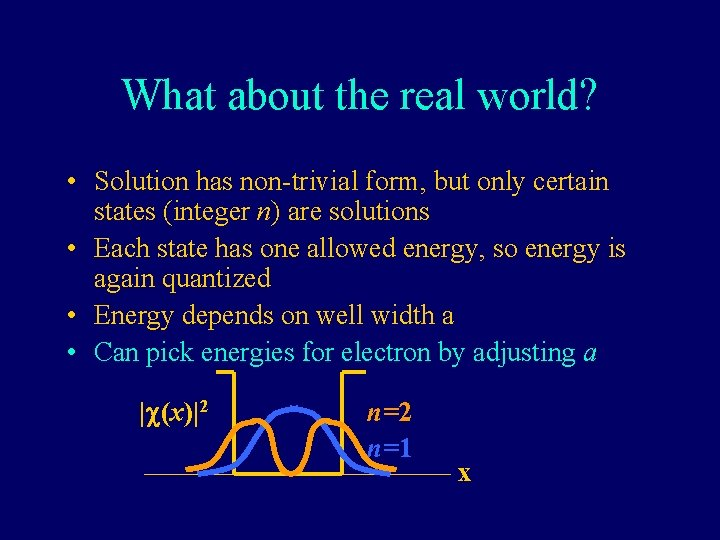What about the real world? • Solution has non-trivial form, but only certain states (integer n) are solutions • Each state has one allowed energy, so energy is again quantized • Energy depends on well width a • Can pick energies for electron by adjusting a |c(x)|2 n=1 xPutting Several Wells Together • How does the number of energy bands compare with the number of energy levels in a single well? • As atom spacing decreases, what happens to energy bands? • What happens when impurities are added?Quantum wells • An electron is trapped since no empty energy states exist on either side of the well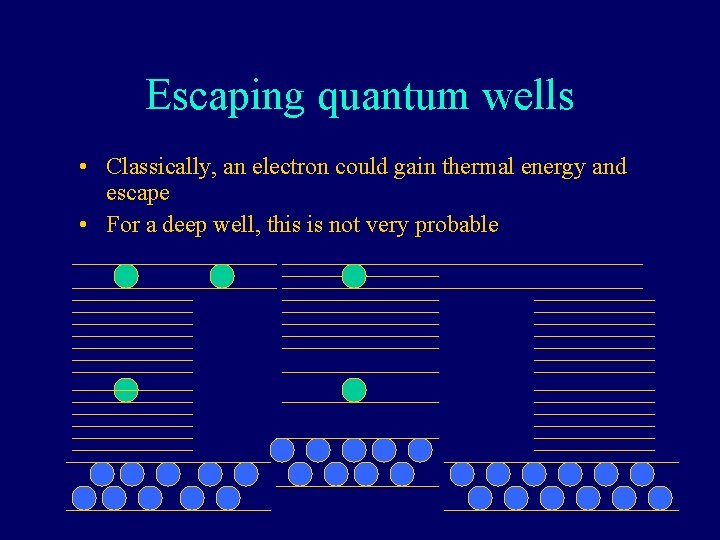Escaping quantum wells • Classically, an electron could gain thermal energy and escape • For a deep well, this is not very probable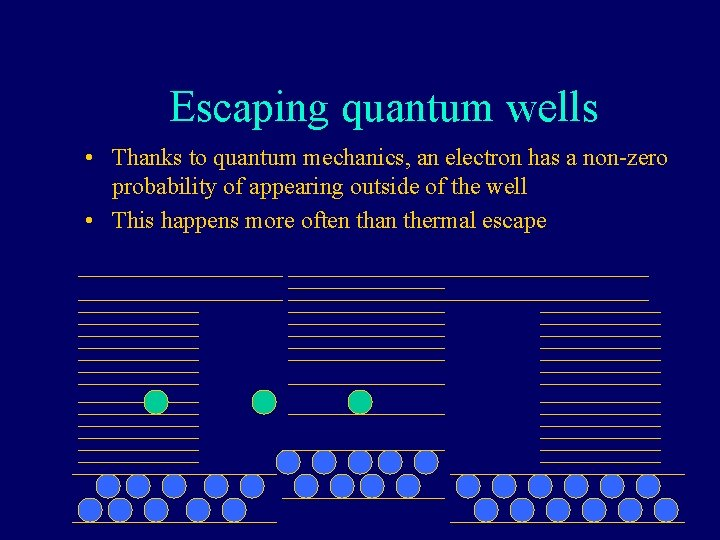Escaping quantum wells • Thanks to quantum mechanics, an electron has a non-zero probability of appearing outside of the well • This happens more often than thermal escapeWhat have we learned today? § Quantum mechanics challenges our physical intuition but it is the way things really work. § Particles are described with a wave function Y(x, t) which describes the propagation through space and time (when unobserved).What have we learned today? § Integrating the square of the wave function over a region gives us the probability of finding the object in that region § A “particle” confined to an infinitely-high box is described by a wave function of a sine. § “particles” in finite wells or in atoms are described by more complicated wave functions § All three situations result in quantized (only certain values allowed) energiesBefore the Next Class • Do Activity 23 Evaluation before next class • Finish Homework 24 • Start Reading Chapters 9 and 10 (3 rd hour exam will cover) • Do Reading Quiz 25 (due before class 25)